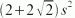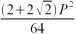SEARCH HOMEMath Central Quandaries & QueriesQuestion from Kathy: If the perimeters are the same, which has the greater area, a circle, a square, an ellipse, or an octagon?Hi Kathy.

Let's use algebra to help with most of this problem:

The area of a circle is πr2 , where r is the radius of the circle. But r = P/(2π), where P is the perimeter of the circle. So the area of the circle is P2 /(4π).

The area of a square is s2 , where s is the side length. But s = P/4, so the area of a square is P2 /16. Since 1/(4π) > (1/16), the circle has more area than the square.

The area of an octagon iswhere s is the side length, but s = P/8. So the area of an octagon isSince this is less than 1/(4π), the circle has more area than the octagon.

The area of an ellipse is much harder to solve. You need to use advanced calculus for this. However, you can think about it this way: If you blow soap bubbles, then nature will make the smallest stable bubble it can around a certain quantity of air. That means that this shape has the largest volume for a given surface area. Of course, the shape is a sphere, not an egg, and the same thing happens in two dimensions: a circle with a given perimeter will never have less area than an ellipse with the same perimeter.

So a circle has more area than an ellipse.

Stephen La Rocque. >Math Central is supported by the University of Regina and The Pacific Institute for the Mathematical Sciences.